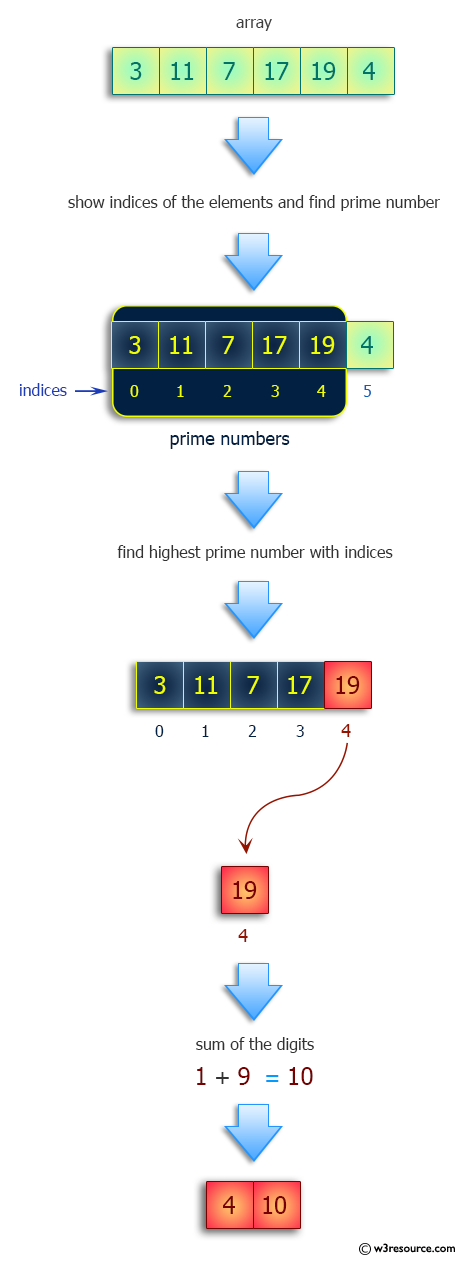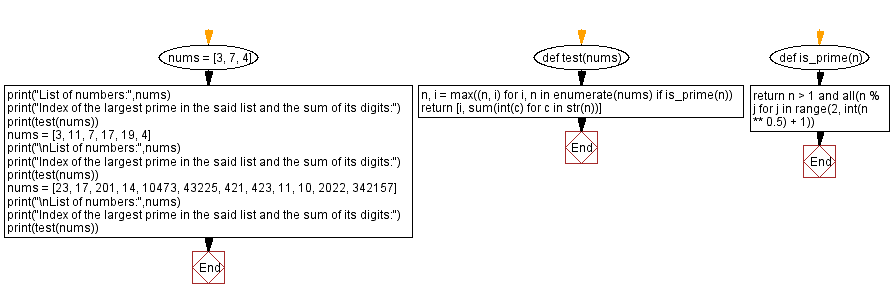﻿ Python: Find the index of the largest prime in the list and the sum of its digits - w3resource# Python: Find the index of the largest prime in the list and the sum of its digits

## Python Programming Puzzles: Exercise-76 with Solution

Write a Python program to find the index of the largest prime in the list and the sum of its digits.

```Input:
[3, 7, 4]
Output:
[1, 7]

Input:
[3, 11, 7, 17, 19, 4]
Output:
[4, 10]

Input:
[23, 17, 201, 14, 10473, 43225, 421, 423, 11, 10, 2022, 342157]
Output:
[6, 7]
```

Pictorial Presentation:Sample Solution:

Python Code:

``````#License: https://bit.ly/3oLErEI

def test(nums):
n, i = max((n, i) for i, n in enumerate(nums) if is_prime(n))
return [i, sum(int(c) for c in str(n))]
def is_prime(n):
return n > 1 and all(n % j for j in range(2, int(n ** 0.5) + 1))

nums = [3, 7, 4]
print("List of numbers:",nums)
print("Index of the largest prime in the said list and the sum of its digits:")
print(test(nums))
nums = [3, 11, 7, 17, 19, 4]
print("\nList of numbers:",nums)
print("Index of the largest prime in the said list and the sum of its digits:")
print(test(nums))
nums = [23, 17, 201, 14, 10473, 43225, 421, 423, 11, 10, 2022, 342157]
print("\nList of numbers:",nums)
print("Index of the largest prime in the said list and the sum of its digits:")
print(test(nums))
``````

Sample Output:

```List of numbers: [3, 7, 4]
Index of the largest prime in the said list and the sum of its digits:
[1, 7]

List of numbers: [3, 11, 7, 17, 19, 4]
Index of the largest prime in the said list and the sum of its digits:
[4, 10]

List of numbers: [23, 17, 201, 14, 10473, 43225, 421, 423, 11, 10, 2022, 342157]
Index of the largest prime in the said list and the sum of its digits:
[6, 7]
```

Flowchart:## Visualize Python code execution:

The following tool visualize what the computer is doing step-by-step as it executes the said program:

Python Code Editor :

Have another way to solve this solution? Contribute your code (and comments) through Disqus.

What is the difficulty level of this exercise?

Test your Programming skills with w3resource's quiz.

﻿

## Python: Tips of the Day

Clamps num within the inclusive range specified by the boundary values x and y:

Example:

```def tips_clamp_num(num,x,y):
return max(min(num, max(x, y)), min(x, y))
print(tips_clamp_num(2, 4, 6))
print(tips_clamp_num(1, -1, -6))
```

Output:

```4
-1
```